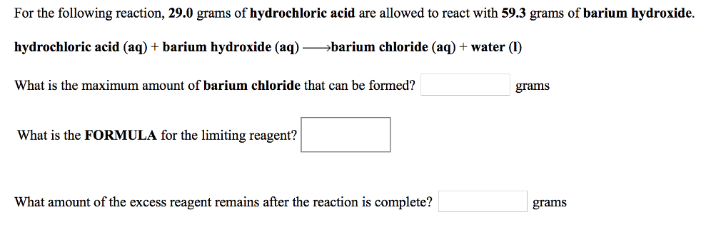# Problem: For the following reaction, 29.0 grams of hydrochloric acid are allowed to react with 59.3 grams of barium hydroxide.hydrochloric acid (aq) + barium hydroxide (aq) → barium chloride (aq) + water (l) What is the maximum amount of barium chloride that can be formed? What is the FORMULA for the limiting reagent? What amount of the excess reagent remains after the reaction is complete?

###### FREE Expert Solution
82% (93 ratings)###### Problem Details

For the following reaction, 29.0 grams of hydrochloric acid are allowed to react with 59.3 grams of barium hydroxide.

hydrochloric acid (aq) + barium hydroxide (aq) → barium chloride (aq) + water (l)

What is the maximum amount of barium chloride that can be formed?

What is the FORMULA for the limiting reagent?

What amount of the excess reagent remains after the reaction is complete?Frequently Asked Questions

What scientific concept do you need to know in order to solve this problem?

Our tutors have indicated that to solve this problem you will need to apply the Limiting Reagent concept. You can view video lessons to learn Limiting Reagent. Or if you need more Limiting Reagent practice, you can also practice Limiting Reagent practice problems.